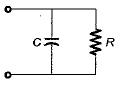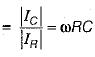Courses

# Frequency Response And Resonance

## 10 Questions MCQ Test Topicwise Question Bank for GATE Electrical Engineering | Frequency Response And Resonance

Description
This mock test of Frequency Response And Resonance for Electrical Engineering (EE) helps you for every Electrical Engineering (EE) entrance exam. This contains 10 Multiple Choice Questions for Electrical Engineering (EE) Frequency Response And Resonance (mcq) to study with solutions a complete question bank. The solved questions answers in this Frequency Response And Resonance quiz give you a good mix of easy questions and tough questions. Electrical Engineering (EE) students definitely take this Frequency Response And Resonance exercise for a better result in the exam. You can find other Frequency Response And Resonance extra questions, long questions & short questions for Electrical Engineering (EE) on EduRev as well by searching above.
QUESTION: 1

### In a double tuned circuit, consisting of two magnetically coupled, identical high Q tuned circuits, at the resonance frequency of either circuit, the amplitude response has

Solution:

Since quaiity factor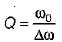and Q is high therefore, the resonant frequency ω0 will be high. Hence, the amplitude response will have a peak always

QUESTION: 2

### A network function contains only poles whose real-parts are zero or negative. The network is

Solution:

Poles are at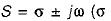can be zero or negative). The system will be stable if the poles at jω-axis are simple.

QUESTION: 3

### The minimum amount of hardware required to make a high pass filter is

Solution: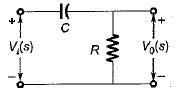Figure above shows a high-pass filter having a transfer function,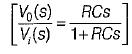QUESTION: 4

The Q-factor of a series RLC circuit having R = 10 Ω , L = 0.01 H and C = 100 μF is

Solution: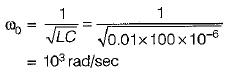Also, bandwidth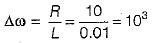∴ Quantity factor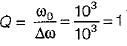QUESTION: 5

The input frequency of the given circuit for unity power factor operation is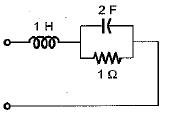Solution:

Input impedance,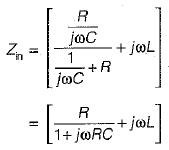or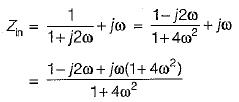For unity p.f. operation, Img(Zin) = 0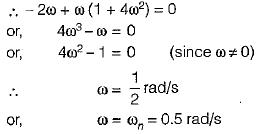QUESTION: 6

When XL is equal to Xc , then

Solution:
QUESTION: 7

If the quality factor of a series resonant circuit is increased then, its selectivity will

Solution:

Q∝  selectivity

QUESTION: 8

The steady state transfer function H(Jω) of the network shown below is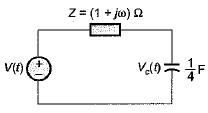Solution:

Transfer function,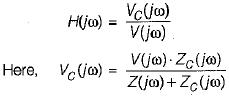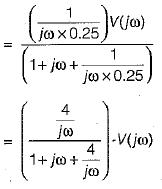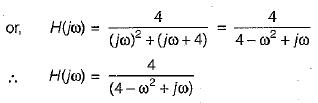QUESTION: 9

The transfer function of the circuit shown below is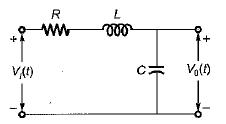Solution: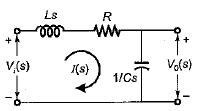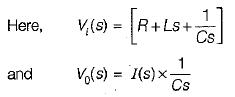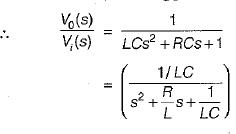QUESTION: 10

The figure of merit for a capacitor in parallel with a resistance R is given by

Solution:

Figure of merit = Q-factor